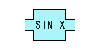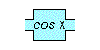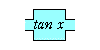Math 115  Lab #5
M. Flashman

I. Graphing Piecewise Defined Functions (demo)
II.
Applets that visualize finding the values for trig functions

• Tools for Winplot used in this lab:
• User Function
• joinx(.....).
• point
• Inventory
• table

I.Graphing Piecewise Defined Functions- Demonstration.
• User Function
• You can build piecewise (spliced) functions, namely joina, joinb, joinc, ..., and joinz.
• For example, the value of

joinx(f(x)|c,g(x)|d,h(x))
is
f(x) if x <= c,
g(x) if c < x <= d,
h(x) otherwise.

For example, try graphing y = joinx(x+1|0,1-xx|2,-1).
• Using this example move the slider and check y for x = -3, x= 1, x = 5.
• Check the values that appear in the table for this function.
• Use point to complete the figure accurately.

II. Applets that visualize finding/estimating the values for trig functions
from http://www.ies.co.jp/math/java/trig/index.htmlSine Function BoxCosine Function BoxTangent Function Box

Record your answers for the next work and submit them on Moodle by Wednesday, Feb. 24

1. For each of the following angles, measured in degrees, use the appropriate function box to find the sine, cosine, and tangent for that angle: [Compare these results with results using your calculator.]
• 35 degrees
• 15 degrees
• 70 degrees
• 50 degrees
2. For each of the following numbers, a, use the appropriate function box to estimate the angle t  measured in degrees so that sin(t) = a. [Compare these results with results using your calculator.]
• a = 0.47
• a = 0.80
• a = 0.32
• a = 0.94

3. For each of the following numbers, b, use the appropriate function box estimate the angle t  measured in degrees so that cos(t) = b. [Compare these results with results using your calculator and the results of problem 1.]
• b = 0.47
• b = 0.80
• b = 0.32
• b = 0.94
4. For each of the following numbers, c, use the appropriate function box estimate the angle t  measured in degrees so that tan(t) = c. [Compare these results with results using your calculator.]
• c = 0.47
• c = 2.80
• c = 0.73
• c = 4.37
5. Use the function boxes to estimate the angle t measured in degrees so that sin(t ) = cos (s) where tan (s) = 2.5.
[Compare this results with a result using your calculator.]

End of Lab 5